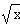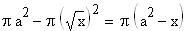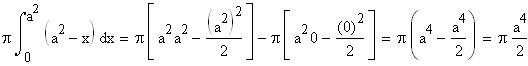Problem
Find the volume of the solid generated by rotating the region R bounded by the y axis, the line y = a, and the curve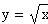around the x axis.
Solution
Problems like this are always easier to solve using pictures. Here is the region R.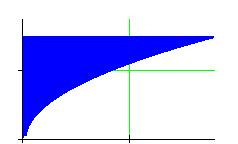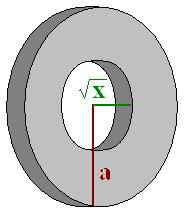If R is rotated around the x axis, the generated solid is a solid cylinder with a parabolic hole scooped in the middle. A horizontal cross section looks like a washer with inside radius